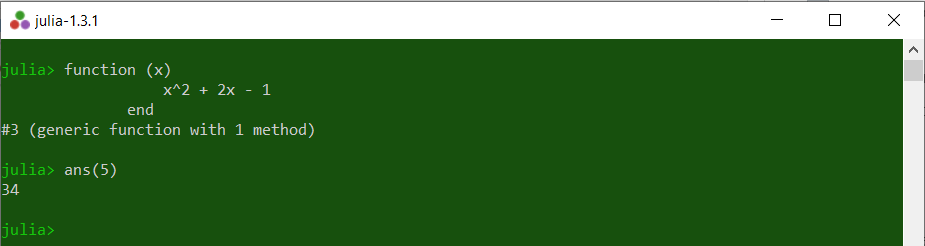Related Articles

# Functions in Julia

• Last Updated : 26 Mar, 2020

A function in Julia is an object that takes a tuple of arguments and maps it to a return value. A function can be pure mathematical or can alter the state of another object in the program.
With Julia’s function, we can write bigger computations but in very fewer lines of code as function support some comprehensive notations. Creating and using functions in Julia is very easy.

#### Defining a function

Functions in Julia can be of multiple types as per the requirement. Some of these types are listed below:

• A function with single expression
• A function with multiple expressions
• A function with no argument
• A function with variable arguments, etc.

Defining a function is as easier as calling a function, just write the predefined keyword `function` and provide a function_name. A function can be assigned with a single expression to follow or multiple expressions or no expression. It is not necessary to provide expressions to some functions to perform operations. For ex- Mathematical addition operator(+): It can just be called with arguments and the function will return the output as addition of those arguments.

Note: Just like looping statements, a function also needs an end statement to mark the closing of the function.

Example:

 `# Defining a function``function fn()``    ``println(``"this is a function"``)``end`` ` `# Function call``fn()`

Output:

```this is a function
```

#### Function with arguments

Functions with arguments can also be created by passing a tuple of arguments. Defining such function is also as above but with a little modification. Also, functions can be assigned with some other names or we can say that function is copied from one name to another.

 `# Defining a function with arguments``function add_fn(x, y)``    ``println(x ``+` `y)``end`` ` `# Assigning another name to function``another_add ``=` `add_fn`` ` `# Calling defined function``add_fn(``7``, ``8``)`` ` `# Calling function by new name``another_add(``6``, ``7``)`

Output:

```15
13
```

#### Shorthand defining a function

A single line function can also be defined using ‘=’(assignment operator). Using such notation is useful as it will save time and lines of source code.

 `# Defining a shorthand function``add_fn(x, y) ``=` `println(x ``+` `y)`` ` `# Function call``add_fn(``7``, ``8``)`

Output:

```15
```

#### Use of Return in a Function

Sometime we want our function to return some value which can further be used for some computation. For returning a value from a function return keyword is used. This will return the computed value as per the instructions defined in the function, to the statement from where the function is being called. A return statement can also be used to compute the operation of the function by just writing the whole operation after the return statement. This will result in fewer lines of code, by not writing the statements to compute and then returning the computed value.

 `# Defining a function``function add_fn(x, y)`` ` `    ``# Defining return statement``    ``return` `x ``+` `y``end`` ` `# Function call``z ``=` `add_fn(``1``, ``9``)``println(z)`

Output:

```10
```

The above code will take values of ‘x’ and ‘y’ as arguments and then return them after adding ‘x’ and ‘y’ and will store the returned result in another variable ‘z’ from where the function is being called.

Note:
Whenever something is returned the function is exited. So the statement after the return statement will not work.

Example:

 `# Defining a function``function fn(x, y)`` ` `    ``# Return statement``    ``return` `x ``*` `y``    ``println(``"This is not executed"``)``end`` ` `# Function call``out ``=` `fn(``7``, ``7``)`` ` `println(out)`

Output:

```49
```

As in the above code, the output is only what is returned and the statement after return is not executed.

#### Skipping the return statement

There is no need to use `return` keyword if we are using only a single statement inside out function. This is because Julia automatically returns the output of the last processed statement in the function.

 `# Defining function``function f(x, y)``    ``x ``*` `x``end``function g()``    ``println(``"printed data is returned"``)``end`` ` `# Function call``out1 ``=` `f(``5``, ``5``)``out2 ``=` `g()`` ` `println(out1)``println(out2)`

Output:

```25
printed data is returned
```

#### Using Operators as functions

In Julia, operators can also be used as a function. Operators like +, -, *, etc are functions. Since operators can work as functions we can alias (give another name) to an operator.

Note: When assigning an operator to a variable, the statement line needs to be ended with a ;(semicolon). Otherwise an Error will be generated.

Example:

 `# Performing addition operation directly``a ``=` `50` `+` `20` `+` `1``println(a)`` ` `# '+' working as function``b ``=` `+``(``50``, ``20``, ``1``)``println(b)`` ` `# aliasing operator as function``f ``=` `+``;``println(f(``50``, ``20``, ``1``))`

Output:

```71
71
71
```

#### Anonymous Functions

Sometimes there is no need to think of a name for a function. Julia allows creating functions without a name as well. Now, a problem arises that how to call these functions or how to pass arguments to these functions. Julia provides a keyword `ans` which automatically refers to the last computed value. This keyword can be used to pass arguments to the anonymous function.

Example:

 `# Creating an anonymous function``function (x)``           ``x^``2` `+` `2x` `-` `1``       ``end`` ` `# Passing argument to function``ans(``5``)`

Output:My Personal Notes arrow_drop_up